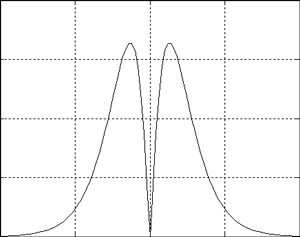DOI: https://doi.org/10.3103/S0735272713060071Open AccessSubscription Access### Class of non-Gaussian distributions with zero skewness and kurtosis

Aleksandr I. Krasil'nikov

#### Abstract

A mathematical model of non-Gaussian distributions with zero skewness and kurtosis has been defined. This model represents a class of two-component mixtures of conjugate distributions with equal weight coefficients. An equation was obtained that the second and fourth initial moments of the mixture components should satisfy. Examples of non-Gaussian distributions with zero skewness and kurtosis were considered. These examples showed that the sixth and eighth cumulant coefficients in such distributions could be positive, negative or nonexistent. The obtained results make it possible to perform the mathematical and computer simulation of non-Gaussian distributions with zero skewness and kurtosis.

#### Keywords

non-Gaussian distribution; coefficient of skewness; coefficient of kurtosis; mathematical simulation; computer simulation; random quantity; characteristic function; cumulant coefficient

#### Full Text:

PDF#### References

NOVITSKII, P.V. AND ZOGRAF, I.A. Error Estimation in Measurement Results. Leningrad: Energoatomizdat, 1991. 304 p. [in Russian].

LEZIN, YU.S. Introduction to the Theory and Technology of Radio Engineering Systems: Textbook for Institutes of Higher Education. Moscow: Radio i Svyaz’, 1986. 280 p. [in Russian].

FOMIN, YA.A. AND TARLOVSKII, T.R. Statistical Theory of Image Recognition. Moscow: Radio i Svyaz’, 1986. 264 p. [in Russian].

LEVIN, B.R. AND SHVARTS, V. Probabilistic Models and Methods in the Communication and Control Systems. Moscow: Radio i Svyaz’, 1985. 312 p. [in Russian].

TIKHONOV, V.I. Statistical Radio Engineering. Moscow: Radio i Svyaz’, 1982. 624 p. [in Russian].

DENISENKO, A.N. Signals. Statistical Radio Engineering. Reference Book. Moscow: Goryachaya Liniya–Telekom, 2005. 704 p. [in Russian].

SHELUKHIN, O.I. AND BELYAKOV, I.V. Non-Gaussian Processes. St. Petersburg: Politekhnika, 1992. 312 p. [in Russian].

HAHN, G.J. AND SHAPIRO, S.S. Statistical Models in Engineering. New York: Wiley-Interscience, 1994.

KENDALL, M. AND STUART, A. Kendall’s Advanced Theory of Statistics. Vol. 1: Distribution Theory, 6th ed., 1998.

LUKACS, E. Characteristic Functions. New York: Hafner, 1970.

KRASIL’NIKOV, A.I. AND PILIPENKO, K.P. Application of two-component Gaussian mixture for identifying unimodal symmetrical probability densities. Elektronika i Svyaz’, n.5(46), p.20-29, 2008.

VADZINSKII, R.N. Probabilistic Distributions: Reference Book. St. Petersburg: Nauka, 2001. 295 p. [in Russian].